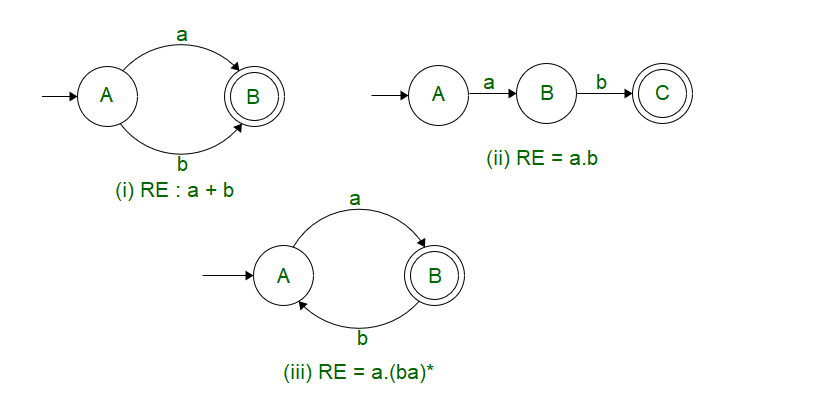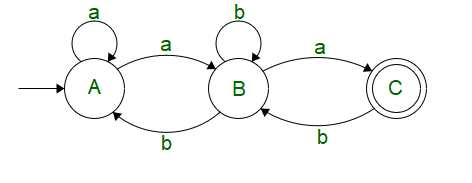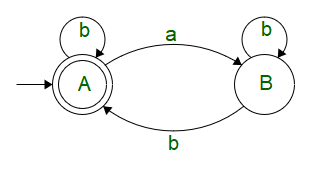# Arden’s Theorem and Challenging Applications | Set 2

Having gained the knowledge of how to draw a basic Finite State Machine ( DFA, NFA or-NFA ). We head to deriving a Regular Expression from the provided state machine.

For certain examples provided below, it’s fairly simple to derive it.But for the following example, it’s fairly hard to derive the Regular Expression by just observing the Finite State Machine. For this purpose, we make use of Arden’s Theorem to simplify our Individual State Equations and come up with our final state equation ( which may or may not be the simplified version )Arden’s Theorem states that, if P & Q are two regular expressions over, and if P does not contain, then the following equation R given by R = Q + RP has a unique solution ; R = QP*

PROOF  :-
R = Q + RP
R = Q + QP*P   ( Substituting the value of R )
R = Q(+ P*P)
R = QP*    ( P*P =,+= P* )

Let’s solve the provided automata above with the help of Arden’s Theorem.

We see, that on state C, there is a state transition coming from B when a is the input

 C = Ba

On state B, There is a self loop on input b, a transition from A when input is a, a transition from state C when input is b

 B = Bb + Cb + Aa

On state A, There is atransition ( being the start state,transition must be included), a self loop on input a, a transition from B when input is b.

 A =+ Aa + Bb

Putting (2) in (1), we get

C = Ba
C = (Aa + Bb + Cb)a
C = Aaa + Bba + Cba

Putting (1) in (2), we get

B = Bb + Cb + Aa
B = Aa + Bb + (Ba)b
B = Aa + B(b + ab)
B = Aa(b + ab)*  (Using R = QP*)

Putting (2) in (3), we get

A =+ Aa + Bb
A =+ Aa + Aa(b + ab)*b
A =+ A(a + a(b + ab)*b)
A =(a + a(b + ab)*b)*
A = (a + a(b + ab)*b)*

As a final step, Let’s combine all the simplified equations onto the final state C

C = Ba
C = Aa(b + ab)*a
C = (a + a(b + ab)*b)* a (b + ab)* a

Now this example corresponded to direct derivation from a provided NFA to a Regular Expression.

Let’s say, we are provided with a problem that goes like

Problem : Derive a regular expression to represent a language having even no. of a’s

For this case, it’s difficult to arrive at a regular expression with just trial and error methodology.
We might come across sample solutions like :-

1. ( aa + b )*
2. ( b*ab* )*
3. (b*ab*ab*)*

which might satisfy some cases, but also leads to unwanted cases and missing cases with alternate a’s and b’s.
The best way to solve this problem is to first draw a finite state machine for the same, and then derive the regular expression from the same.

The figure below shows the DFA for the provided problemNow that we have the DFA, let’s solve it using Arden’s Theorem of Individual State Equations.

We see that on state A, there is a self loop with input b and transition from B with input a

A =+ Ab + Ba

We see that on state B, there is a self loop on input b and transition from A when input is a.

B = Aa + Bb

Taking equation for B, we can apply Arden’s theorem

B = Aa + Bb
B = Aab*

Substituting the value of B in A we get

A =+ Ab + Ba
A =+ Ab + (Aab*)a
A =( b + ab*a )*
A = ( b + ab*a )*

Hence, the regular expression for the provided problem is RE : ( b + ab*a )*

We see that Arden’s theorem can be used as a powerful simplification tool to determine the Regular Expressions and also to design the desired Finite State Machine from the same.

Don’t stop now and take your learning to the next level. Learn all the important concepts of Data Structures and Algorithms with the help of the most trusted course: DSA Self Paced. Become industry ready at a student-friendly price.

My Personal Notes arrow_drop_upCheck out this Author's contributed articles.

If you like GeeksforGeeks and would like to contribute, you can also write an article using contribute.geeksforgeeks.org or mail your article to contribute@geeksforgeeks.org. See your article appearing on the GeeksforGeeks main page and help other Geeks.

Please Improve this article if you find anything incorrect by clicking on the "Improve Article" button below.

9

Please write to us at contribute@geeksforgeeks.org to report any issue with the above content.